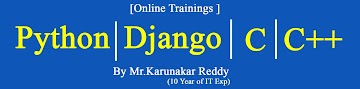-->### Microsoft-Excel 2007 Study Material Day-Twenty Two

STATISTICAL FUNCTIONS :

1)AVERAGE :Returns the average (arithmetic mean) of the arguments.
Syntax : =AVERAGE(number1, [number2], ...)
Example  :  =AVERAGE(10,20,30,40,50)  (OR)  =AVERAGE(A1:D1)

2)MAX : Returns the largest value in a set of values.
Syntax : =MAX(number1, [number2], ...)
Example : =max(2,6,8,4)   (or) =MAX(A1:A10)

3)MIN : Returns the smallest number in a set of values.
Syntax : =MIN(number1, [number2], ...)
Example : =MIN(4,9,21,35,8) (or) =MIN(A1:A10)

4)COUNT : The COUNT function counts the number of cells that contain numbers, and counts numbers within the list of arguments.
Syntax : =COUNT(RANGE)
Example : =COUNT(A1:A10)

5)COUNT BLANK :Counts empty cells in a specified range of cells.
Syntax : =COUNTBLANK(RANGE)
Example : COUNT(A1:A10)

6) MEDIAN :Returns the median of the given numbers. The median is the number in the middle of a set of numbers.
Syntax : MEDIAN(number1, [number2], ...)
Example : =Median(1,2,3,5,6)  (or) =Median(a1:a10)